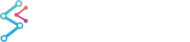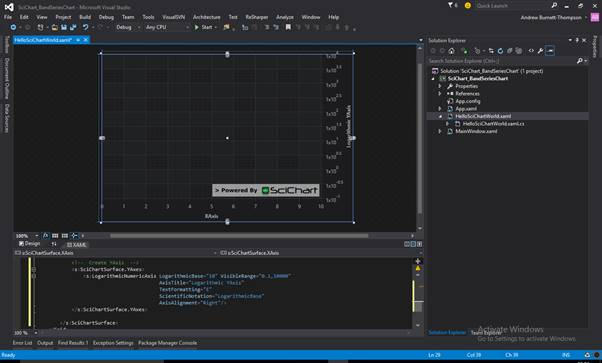﻿ Axis Types in SciChart | WPF Chart DocumentationSciChart WPF 2D Charts > Axis APIs > Axis Types in SciChart
Axis Types in SciChart

## NumericAxis

The NumericAxis is a Value-Axis and is suitable for X and Y Axis when the data on that axis is numeric (e.g. double, int, long, float, short). It is not suitable for TX=DateTime or TimeSpan.

### Declaring a NumericAxis in XAML

Declaring a NumericAxis can be done in XAML or in code

Declaring a NumericAxis
Copy Code
```<s:SciChartSurface>
<!-- ... omitted for brevity -->
<!--  Create a NumericAxis  on the YAxis -->
<s:SciChartSurface.YAxis>
<s:NumericAxis AxisTitle="Number of Samples (per Series)" TextFormatting="n2" AutoRange="Once" VisibleRange="0,10" GrowBy="0.1, 0.1" AxisAlignment="Left"/>
</s:SciChartSurface.YAxis>
<!-- ... omitted for brevity -->
</s:SciChartSurface>
```

### Declaring a NumericAxis in Code

Declaring a NumericAxis
Copy Code
```var sciChartSurface = new SciChartSurface();
var yAxis = new NumericAxis()
{
TextFormatting = "n2",
AutoRange = AutoRange.Once,
VisibleRange = new DoubleRange(0, 10),
GrowBy = new DoubleRange(0.1, 0.1),
AxisAlignment = AxisAlignment.Left,
AxisTitle = "Number of Samples (per Series)"
};
sciChartSurface.YAxis = yAxis;
```

## DateTimeAxis

The DateTimeAxis is a Value-Axis suitable for the XAxis when the data on the XAxis is a DateTime. It is not suitable for YAxis or other data-types.

### Declaring a DateTimeAxis in XAML

Declaring a DateTimeAxis
Copy Code
```<s:SciChartSurface>
<!-- ... omitted for brevity -->
<!--  Create a DateTimeAxis  on the XAxis -->
<s:SciChartSurface.XAxis>
<s:DateTimeAxis AxisTitle="Time" TextFormatting="dd-MMM-yyyy"
SubDayTextFormatting="HH:mm:ss"
AutoRange="Once" GrowBy="0.0, 0.1"
AxisAlignment="Bottom"/>
</s:SciChartSurface.XAxis>
<!-- ... omitted for brevity -->
</s:SciChartSurface>
```

### Declaring DateTimeAxis in Code

Declaring a DateTimeAxis
Copy Code
```var sciChartSurface = new SciChartSurface();
var xAxis = new DateTimeAxis()
{
TextFormatting = "dd-MMM-yyyy",
SubDayTextFormatting = "HH:mm:ss"
AutoRange = AutoRange.Once,
GrowBy = new DoubleRange(0.0, 0.1),
AxisAlignment = AxisAlignment.Bottom,
AxisTitle = "Time"
};
sciChartSurface.XAxis = xAxis;
```

## TimeSpanAxis

The TimeSpanAxis is a Value-Axis suitable for the XAxis when the data on the XAxis is a TimeSpan. It is not suitable for YAxis or other data-types.

### Declaring a TimeSpanAxis in XAML

Declaring a TimeSpanAxis
Copy Code
```<s:SciChartSurface>
<!-- ... omitted for brevity -->
<!--  Create a DateTimeAxis  on the XAxis -->
<s:SciChartSurface.XAxis>
<s:TimeSpanAxis AxisTitle="Time" TextFormatting="HH:mm:ss.fff"
AutoRange="Once" GrowBy="0.0, 0.1"
AxisAlignment="Bottom"/>
</s:SciChartSurface.XAxis>
<!-- ... omitted for brevity -->
</s:SciChartSurface>
```

### Declaring a TimeSpanAxis in Code

Declaring a TimeSpanAxis
Copy Code
```var sciChartSurface = new SciChartSurface();
var xAxis = new TimeSpanAxis()
{
TextFormatting = "hh\:mm\:ss",
AutoRange = AutoRange.Once,
VisibleRange = new TimeSpanRange(TimeSpan.Zero, TimeSpan.FromMilliseconds(1000),
GrowBy = new DoubleRange(0.0, 0.1),
AxisAlignment = AxisAlignment.Bottom,
AxisTitle = "Time"
};
sciChartSurface.XAxis = xAxis;
```

## CategoryDateTimeAxis for Stock Charts

The CategoryDateTimeAxis is a Category-XAxis and is suitable for the XAxis when the data on the XAxis is a DateTime. It is not suitable for YAxis or other data-types.

#### Declaring a CategoryDateTimeAxis in XAML

Declaring a CategoryDateTimeAxis
Copy Code
```<!--  Create XAxis  -->
<s:SciChartSurface.XAxis>
<s:CategoryDateTimeAxis AxisTitle="Category DateTimeAxis" BarTimeFrame="900" GrowBy="0,0.1"/>
</s:SciChartSurface.XAxis>
```

### Declaring a CategoryDateTimeAxis in Code

Declaring a CategoryDateTimeAxis
Copy Code
```var sciChartSurface = new SciChartSurface();
var xAxis = new CategoryDateTimeAxis()
{
VisibleRange = new IndexRange(0, 1000),
GrowBy = new DoubleRange(0, 0.1),
AxisTitle = "Category DateTimeAxis",
AxisAlignment = AxisAlignment.Bottom,
BarTimeFrame = 15*60,
};
sciChartSurface.XAxis = xAxis;
```

## LogarithmicNumericAxis

The LogarithmicNumericAxis is a Value-Axis with a configurable logarithmic base (e.g. Base 2, E, 10) and is suitable for the XAxis and YAxis when the data on that axis is numeric (e.g. double, int, float, long, short).

#### Declaring a LogarithmicNumericAxis in XAML

Declaring a LogarithmicNumericAxis
Copy Code
```<!--  Create YAxis  -->
<s:SciChartSurface.YAxis>
<s:LogarithmicNumericAxis LogarithmicBase="10" VisibleRange="0.1,10000"
AxisTitle="Logarithmic YAxis"
TextFormatting="E"
ScientificNotation="LogarithmicBase"
AxisAlignment="Right"/>
</s:SciChartSurface.YAxis>
```

### Declaring a LogarithmicNumericAxis in Code

Declaring a LogarithmicNumericAxis
Copy Code
```var sciChartSurface = new SciChartSurface();
var yAxis = new LogarithmicNumericAxis()
{
LogarithmicBase = 10.0,
ScientificNotation = ScientificNotation.LogarithmicBase,
TextFormatting = "E",
VisibleRange = new DoubleRange(0.1, 10000.0),
AxisTitle = "Logarithmic YAxis",
AxisAlignment = AxisAlignment.Right
};
sciChartSurface.YAxis = yAxis;
```## CategoryNumericAxis

The CategoryNumericAxis is a Category-XAxis and is suitable for the XAxis when the data on the XAxis is numeric (e.g. double, int, long, float, short). It is not suitable for TX=DateTime or TimeSpan.

### Declaring a CategoryNumericAxis in XAML

Declaring a CategoryNumericAxis
Copy Code
```<!--  Create XAxis  -->
<s:SciChartSurface.XAxis>
<s:CategoryNumericAxis AxisTitle="CategoryNumericAxis" GrowBy="0,0.1"/>
</s:SciChartSurface.XAxis>
```

### Declaring a CategoryNumericAxis in Code

Declaring a CategoryNumericAxis
Copy Code
```var xAxis = new CategoryNumericAxis()
{
VisibleRange = new IndexRange(0, 1000),
GrowBy = new DoubleRange(0, 0.1),
AxisTitle = "CategoryNumericAxis",
AxisAlignment = AxisAlignment.Bottom,
};
sciChartSurface.XAxis = xAxis;
```

## Discontinuous DateTime Axis and Double-Scale Axis

The DiscontinuousDateTimeAxis is a Value-Axis suitable for the XAxis when the data on the XAxis is a DateTime. It is not suitable for YAxis or other data-types.

This axis differs from the DateTimeAxis in that it allows you to skip periodic dates/times, or time ranges from the chart.

More details about this Axis type can be found in a dedicated Discontinuous DateTime Axis and Double-Scale Axis article.